Function Repository Resource:

# TwinPrimes

Get the pairs of primes that differ by 2

Contributed by: Wolfram Staff
 ResourceFunction["TwinPrimes"][n] gives the first n pairs of primes that differ by 2.

## Examples

### Basic Examples (3)

Here are the first 10 pairs of twin primes:

 In:=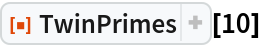Out=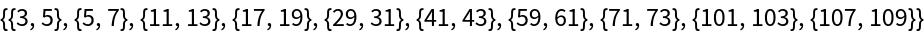Indeed, the differences are 2:

 In:=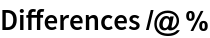Out=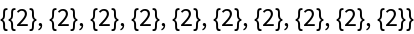For reference, here are the first 30 primes:

 In:=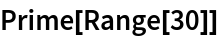Out=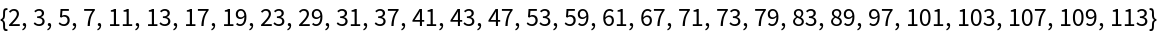### Neat Examples (3)

The sum of the reciprocals of the primes diverges:

 In:=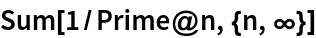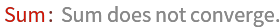Out=In 1919, Brun proved that the sum of the reciprocals of the twin primes converges. The sum, Brun's constant, is around 1.9. Here is the sum of the reciprocals of the first 100,000 twin primes:

 In:=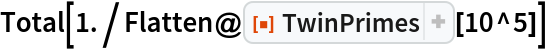Out=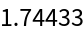This is the last pair in that sum:

 In:=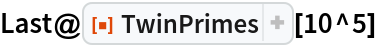Out=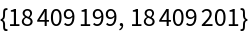## Version History

• 2.0.0 – 05 July 2019
• 1.0.0 – 25 February 2019